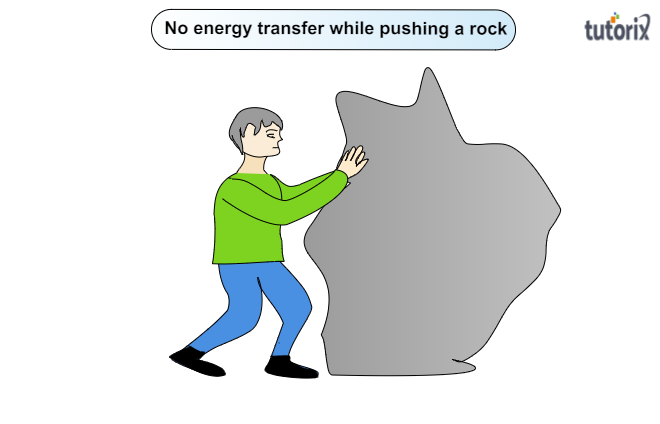# Does the transfer of energy take place when you push a huge rock with all your might and fail to move it? Where is the energy you spend going?

To do:

To determine whether the transfer of energy takes place when a huge rock is pushed with all the might one has and fails to move it. And also we have to check where the spent energy is going.

Solution:

To determine whether the energy transfer is taking place or not while a person gets failed to move a rock despite pushing the rock with all the might, let us know what is the energy transfer:

Energy transfer:

When an object is displaced by applying force, it is called work done or energy transfer.

Formula for the work done:

$\boxed{W=Fd}$

Here $F\rightarrow$ force acted upon the object

$d\rightarrow$ displacement of the object

Energy transfer when the rock does not move:

In this case, there is no displacement.

On using the formula for the work done, $\boxed{W=Fd}$

The work done, $W=Fd=F\times 0$     [here $d=0$, because there is no displacement]

So, there is no energy from the person is transferred to the rock as the rock stays still and consequently gains no kinetic energy.Now let us check where the spent energy is going.

Conversion of muscular energy to heat energy:

Whenever any person attempts to push a huge rock but fails to move it, then no energy of the person is transferred to the rock as it stays still. All things considered, the muscular energy of an individual is converted into heat energy which warms up the individual's body. In this way, he gets exhausted.

Updated on: 10-Oct-2022

113 Views